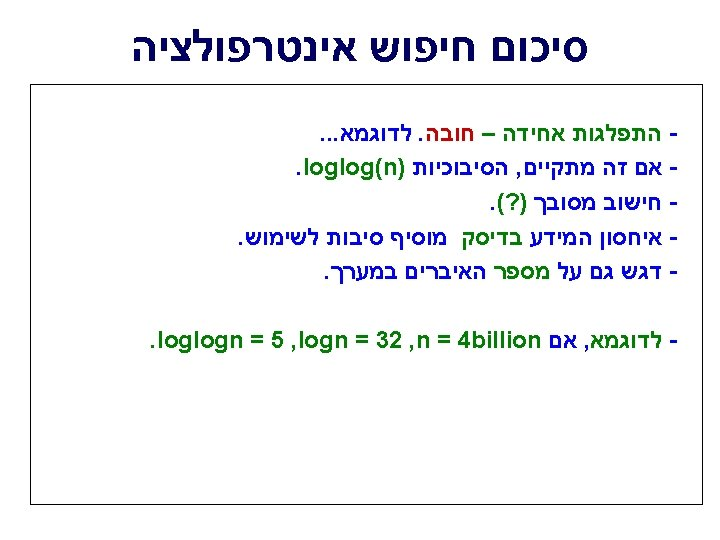# int Sequential Search int k in A int

• Slides: 14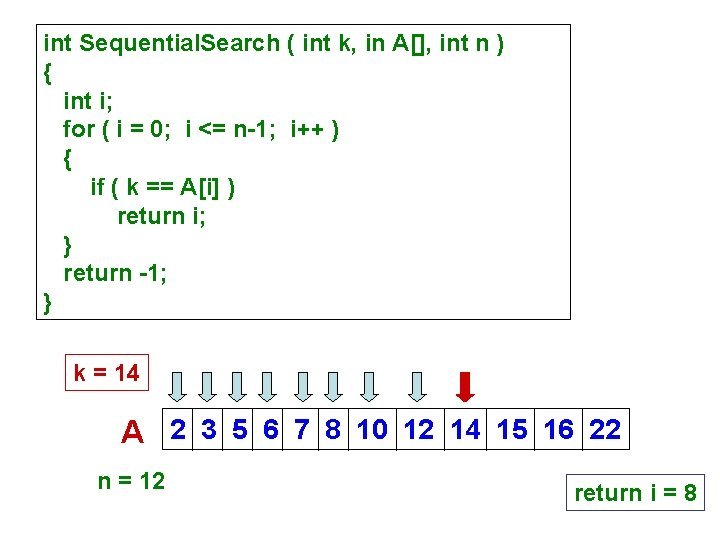int Sequential. Search ( int k, in A[], int n ) { int i; for ( i = 0; i <= n-1; i++ ) { if ( k == A[i] ) return i; } return -1; } k = 14 A 2 3 5 6 7 8 10 12 14 15 16 22 n = 12 return i = 8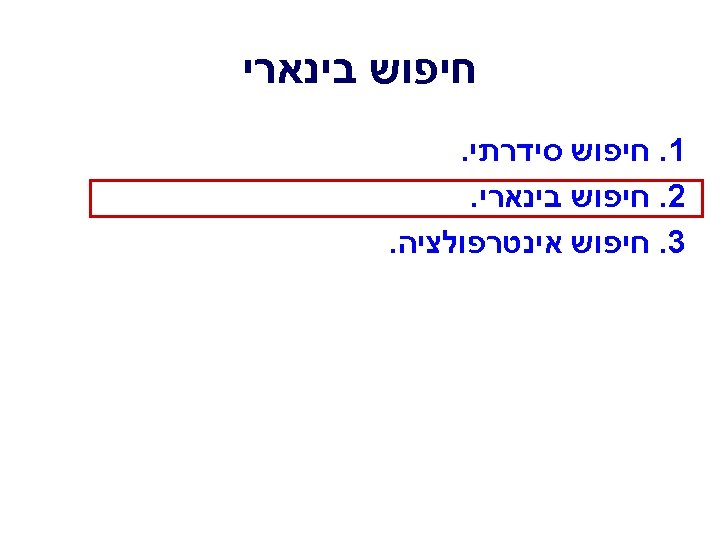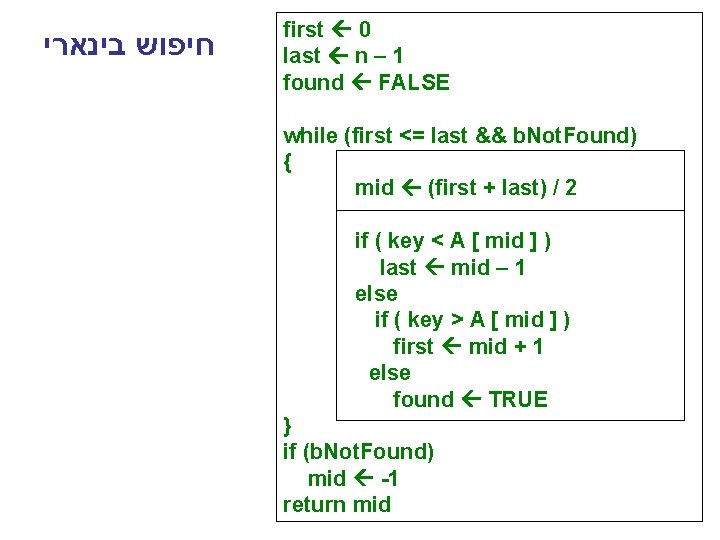חיפוש בינארי first 0 last n – 1 found FALSE while (first <= last && b. Not. Found) { mid (first + last) / 2 if ( key < A [ mid ] ) last mid – 1 else if ( key > A [ mid ] ) first mid + 1 else found TRUE } if (b. Not. Found) mid -1 return midA[], n = 7 k = ‘K’ first 0 last n – 1 found FALSE while (first <= last && b. Not. Found) { mid (first + last) / 2 if ( key < A [ mid ] ) last mid – 1 else if ( key > A [ mid ] ) first mid + 1 else found TRUE } if (b. Not. Found) mid -1 return mid A: ‘B’ ‘E’ ‘F’ ‘H’ ‘K’ ‘N’ ‘S’ index: 0 1 2 3 first 4 5 mid 6 last A: ‘B’ ‘E’ ‘F’ ‘H’ ‘K’ ‘N’ ‘S’ index: 0 1 2 3 4 5 6 first last mid A: ‘B’ ‘E’ ‘F’ ‘H’ ‘K’ ‘N’ ‘S’ index: 0 1 2 3 4 5 first, mid, last 6k = ‘L’ A: ‘B’ ‘E’ ‘F’ ‘H’ ‘K’ ‘N’ ‘S’ first A: mid last ‘B’ ‘E’ ‘F’ ‘H’ ‘K’ ‘N’ ‘S’ first last mid A: ‘B’ ‘E’ ‘F’ ‘H’ ‘K’ ‘N’ ‘S’ first, mid, last A: ‘B’ ‘E’ ‘F’ ‘H’ ‘K’ ‘N’ ‘S’ while (first <= last AND b. Not. Found) last first b. Not. Found return -1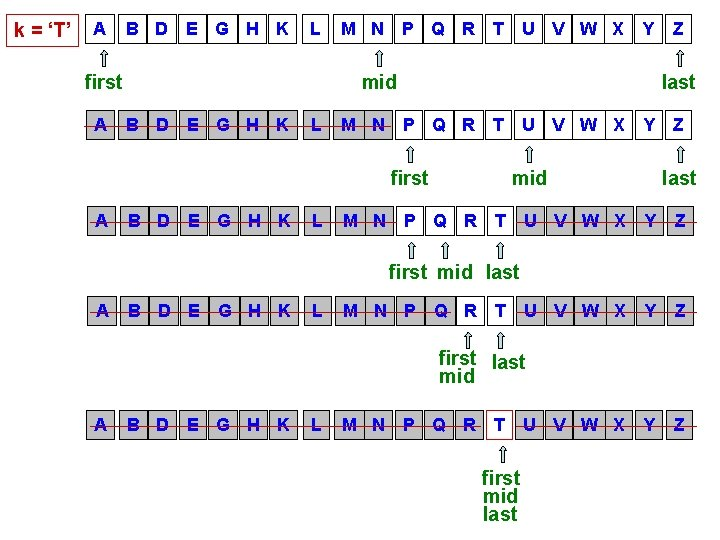k = ‘T’ A B D E G H K L first A M N P Q R T Y mid B D E G H K L M N Z last P Q R T first A U V W X Y mid P Q R T Z last U V W X Y Z Y Z first mid last A B D E G H K L M N P Q R T first last mid A B D E G H K L M N P Q R T first mid last U V W X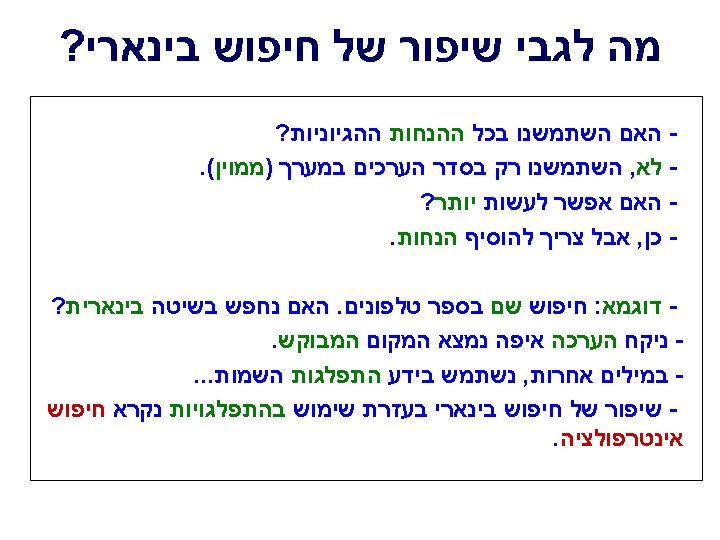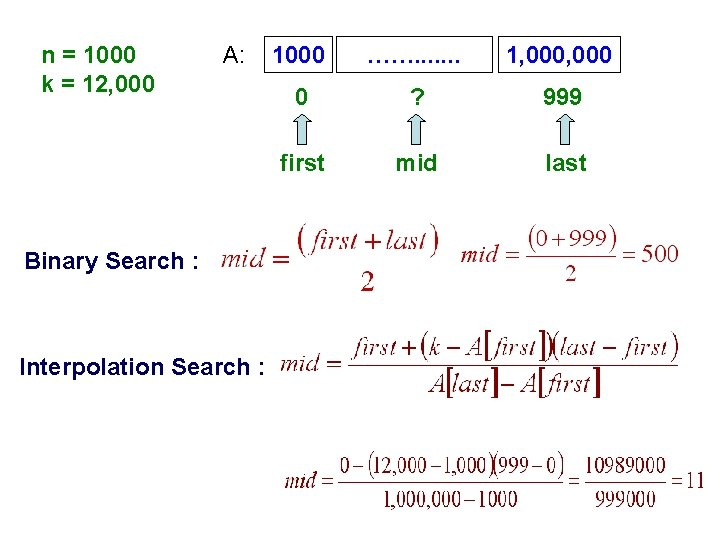n = 1000 k = 12, 000 A: Binary Search : Interpolation Search : 1000 ……. . . . 1, 000 0 ? 999 first mid last